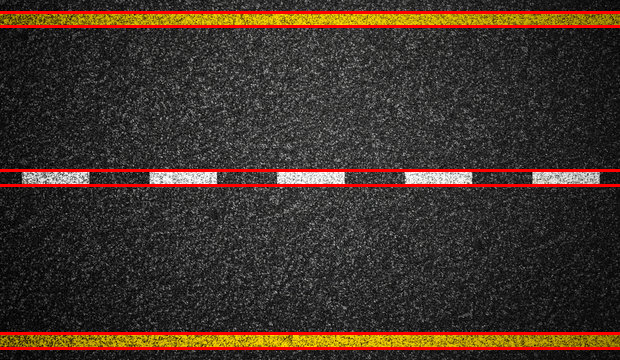• # Line Detection

In this tutorial we’ll learn about line detection using OpenCV. We will use the hough transform method to detect lines.

# What is Hough Transform in OpenCV?

• The Hough transform is a feature extraction technique used in computer vision to detect simple geometric shapes.

• The Hough transform works by converting image data from the Cartesian coordinate system (x,y) to a parameter space represented by a polar coordinate system (r,θ).

• Each point in the image is transformed into a curve in the parameter space, and the intersection of these curves indicates the presence of a particular geometric shape in the image.

• the Hough transform for line detection can be performed using the HoughLines() function

• The output of the function is an array of detected lines in the format (ρ,θ), where ρ is the distance from the origin to the line and θ is the angle between the x-axis and the perpendicular line to the detected line.

InputCode:

``````import cv2
import numpy as np
gray = cv2.cvtColor(img, cv2.COLOR_BGR2GRAY)
edges = cv2.Canny(gray, 50, 150)
lines = cv2.HoughLines(edges, 1, np.pi/180, 300)
for line in lines:
x, y = line
a = np.cos(theta)
b = np.sin(theta)
x0 = a * rho
y0 = b * rho
x1 = int(x0 + 1000 * (-b))
y1 = int(y0 + 1000 * (a))
x2 = int(x0 - 1000 * (-b))
y2 = int(y0 - 1000 * (a))
cv2.line(img, (x1, y1), (x2, y2), (0, 0, 255), 2)
cv2.imshow('Lines', img)
cv2.waitKey(0)
cv2.destroyAllWindows()
``````
• First we imported cv2 and numpy.

• Then, we used cv2.imread() to read the image and cv2.cvtcolor() to convert the image into grayscale.

• Then, we used the canny edge detector to detect edges.

• After this we used the hough transform with a distance resolution of 1 pixel and 300 as a threshold value.

• Then, we used a for loop over lines to extract the line parameters x and y. it calculates the cosine and sine of y, and calculates the (x0, y0) coordinates of the line. Then, it calculates (x1, y1) and (x2, y2) coordinates of the line based on its orientation and length,

• Then, we used cv2.line() to draw the lines on the original image.

• At last, we used cv2.imshow function to display the final result.

Result: